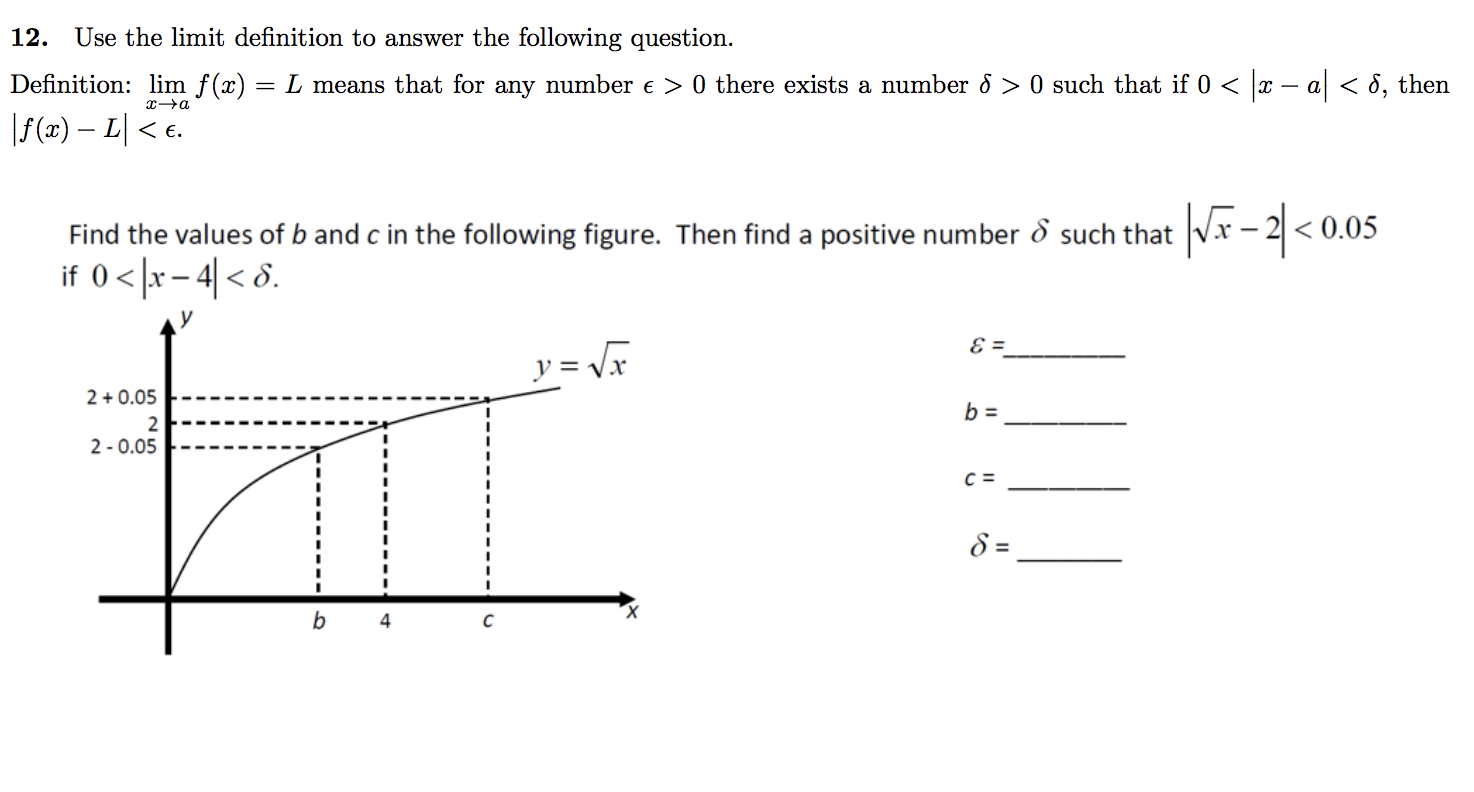# 12. Use the limit definition to answer the following question. Definition: imf x-L means that for any number e> 0 there exists a number δ 〉 0 such that if 0 < x a < δ then Find the values of b and cin the following figure. Then find a positive number δ such that 2 < 0.05 y= x 2-0.05 4

Questionhelp_outlineImage Transcriptionclose12. Use the limit definition to answer the following question. Definition: imf x-L means that for any number e> 0 there exists a number δ 〉 0 such that if 0 < x a < δ then Find the values of b and cin the following figure. Then find a positive number δ such that 2 < 0.05 y= x 2-0.05 4 fullscreen

### Want to see this answer and more?

Experts are waiting 24/7 to provide step-by-step solutions in as fast as 30 minutes!*

*Response times may vary by subject and question complexity. Median response time is 34 minutes for paid subscribers and may be longer for promotional offers.
Tagged in
Math
Calculus

### Limits